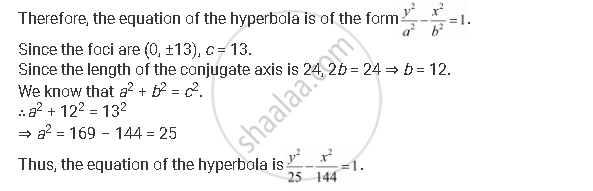Share

# Find the Equation of the Hyperbola Satisfying the Give Conditions: Foci (0, ±13), the Conjugate Axis is of Length 24. - Mathematics

#### Question

Find the equation of the hyperbola satisfying the give conditions: Foci (0, ±13), the conjugate axis is of length 24.

#### Solution

Foci (0, ±13), the conjugate axis is of length 24.

Here, the foci are on the y-axis.Is there an error in this question or solution?